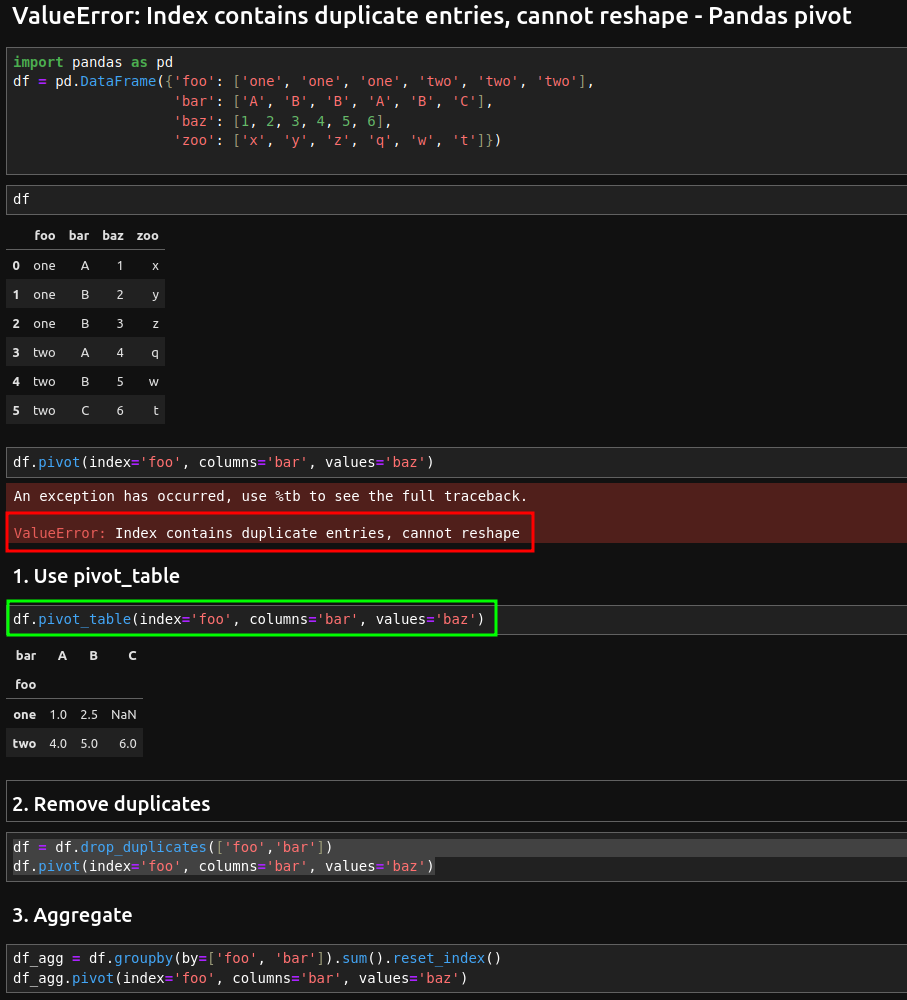In this article we will see how to solve Pandas pivot error: "ValueError: Index contains duplicate entries, cannot reshape".

Let's see how to solve this error in different ways depending on the case.

## Setup

Suppose we have a DataFrame like:

``````import pandas as pd
df = pd.DataFrame({'foo': ['one', 'one', 'one', 'two', 'two', 'two'],
'bar': ['A', 'B', 'B', 'A', 'B', 'C'],
'baz': [1, 2, 3, 4, 5, 6],
'zoo': ['x', 'y', 'z', 'q', 'w', 't']})
``````

You can see data below:

foo bar baz zoo
0 one A 1 x
1 one B 2 y
2 one B 3 z
3 two A 4 q
4 two B 5 w
5 two C 6 t

Note that there is a duplication in the row with index - 2 we have B instead of C.

If we try to use method 'pivot' with duplicate entries like:

``````df.pivot(index='foo', columns='bar', values='baz')
``````

we will get the error:

`ValueError: Index contains duplicate entries, cannot reshape"`## 1. Use pivot_table

For tables with duplicate entries we need to use `pivot_table`:

``````df.pivot_table(index='foo', columns='bar', values='baz')
``````

this will solve the error and produce correct result:

bar A B C
foo
one 1.0 2.5 NaN
two 4.0 5.0 6.0

## 2. Remove duplicates

If you prefer to use the `pivot` method you need to drop duplicates from the DataFrame by:

``````df = df.drop_duplicates(['foo','bar'])
df.pivot(index='foo', columns='bar', values='baz')
``````

In this case the result is the same as using `pivot_table`:

bar A B C
foo
one 1.0 2.5 NaN
two 4.0 5.0 6.0

## 3. Aggregate

You can also use a custom aggregation to mimic pivot behavior. Let's combine methods like:

• `groupby`
• `sum`

to produce aggregate data as the method `pivot` without getting error:

``````df_agg = df.groupby(by=['foo', 'bar']).sum().reset_index()
df_agg.pivot(index='foo', columns='bar', values='baz')
``````

And again we get the same result:

bar A B C
foo
one 1.0 2.5 NaN
two 4.0 5.0 6.0

## Conclusion

In this post, we covered the most common solution for Pandas error on method pivot:

"ValueError: Index contains duplicate entries, cannot reshape".

To solve Pandas errors you need to:

• understand your data very well
• know what the expected result should be.